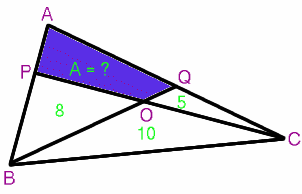# Old Versions of NRICH Problems

##### Age 11 to 18 Challenge Level:

At the BCME conference in April 2018, Charlie and Alison shared some NRICH resources which have been redeveloped to add scaffolding and make them accessible to a wider audience, without reducing the mathematical challenge and complexity.

Original version of Counting Factors

The list below shows the first ten numbers together with their divisors (factors):

1. $1$
2. $1$, $2$
3. $1$, $3$
4. $1$, $2$, $4$
5. $1$, $5$
6. $1$, $2$, $3$, $6$
7. $1$, $7$
8. $1$, $2$, $4$, $8$
9. $1$, $3$, $9$
10. $1$, $2$, $5$, $10$

What is the smallest number with exactly twelve divisors?

What is the smallest number with exactly fourteen divisors?

Original version of Iff

Prove that if n is a triangular number then 8n+1 is a square number. Prove, conversely, that if 8n+1 is a square number then n is a triangular number.

Original version of Areas and RatiosIn the triangle ABC, not drawn to scale, the areas of the smaller triangles are shown. What is the area of the quadrilateral APOQ?